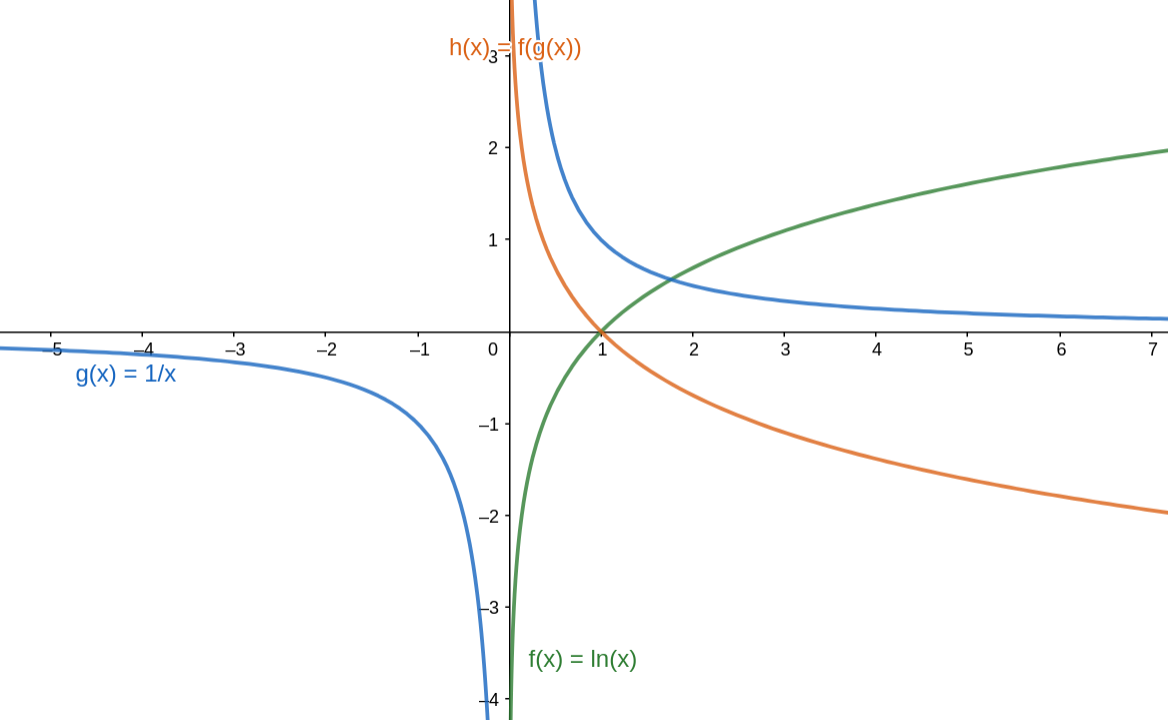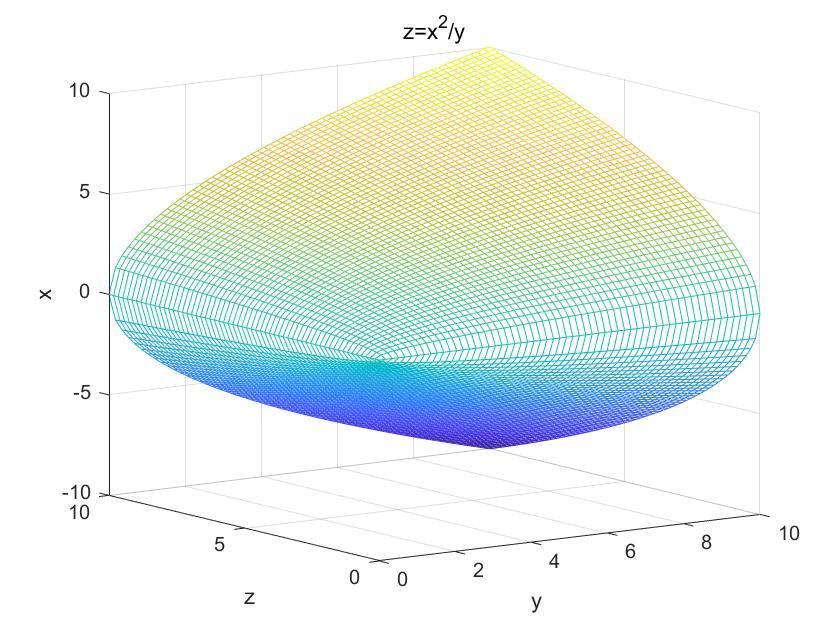• 似然函数，在机器学习的算法模型中，可谓是屡见不鲜了，每次总觉得自己已经掌握了这个概念，但是遇到具体的情况后，发现还是很难说清楚，于是根据wiki上关于Likelihood function的解释，以及个人的学习理解，整理...


文章目录
1. 似然函数与概率1.1 似然函数与概率的初步认识1.2 似然的定义1.3 结合具体实例来深入理解似然与概率
2. 极大似然估计3. 对数似然函数4. 总结

1. 似然函数与概率
1.1 似然函数与概率的初步认识
似然函数，在机器学习的算法模型中，可以说是“老熟人”了，虽然总感觉自己已经掌握了这个概念，但是每次遇到具体的情况时，发现还是难以说清楚，于是根据wiki上关于Likelihood function的解释，以及个人的学习理解，整理笔记如下：
在统计学中，似然函数（或简称似然）是一个基于给定数据模型参数的特殊函数，似然(likelihood)与概率(probability)所表示的意义是完全不同的，具体如下：

在给定参数值的情况下，概率用于描述未来出现某种情况的观测数据的可信度。 在给定观测数据的情况下，似然用于描述参数值的可信度。

听起来可能会觉得摸不着头脑，先不着急，待我给出似然的定义之后，我们再来结合具体实例来理解上面的两句话。
1.2 似然的定义
根据概率的分布属于离散型还是连续型，似然函数的定义也略有不同，下面分别进行说明：
（1）对于离散型变量
假设X是一个离散型的随机变量，它的概率分布p取决于参数θ，那么它的似然函数定义为：

L

(

θ

∣

x

)

=

p

θ

(

x

)

=

P

θ

(

X

=

x

)

=

P

(

X

=

x

∣

θ

)

=

P

(

X

=

x

;

θ

)

L(\theta | x)=p_{\theta}(x)=P_{\theta}(X=x)=P(X=x | \theta)=P(X=x ; \theta)

【注】其中

L

(

θ

∣

x

)

L(\theta | x)

为

L

(

Θ

=

θ

∣

X

=

x

)

L(\Theta=\theta | X=x)

的缩写，它表示基于给定的X=x，我们认为参数Θ=θ的似然（可信度），它的值则等于基于给定的参数Θ=θ，我们预测出现X=x的概率（可信度）（概率有好几种表达方式，这里全部列举了出来…）
（2）对于连续型变量
假设X是一个连续型的随机变量，它的概率分布是一个概率密度函数f（f取决于参数θ），这时它的似然函数定义为：

L

(

θ

∣

x

)

=

f

θ

(

x

)

{L}(\theta |{x})={f}_{\theta}({x})

【注】

L

(

θ

∣

x

)

L(\theta | x)

的含义与上面一致，不做解释,

f

θ

(

x

)

{f}_{\theta}({x})

表示基于参数 θ ，预计 X=x 的概率值为

f

θ

(

x

)

{f}_{\theta}({x})

，也可写作

f

(

x

∣

θ

)

{f}({x} | \theta)

。这个看着有点抽象，举个具体实例来进行说明。        比如说X服从正态分布，我们知道正态分布的概率密度函数形式是：

f

(

x

)

=

1

σ

2

π

e

−

(

x

−

μ

)

2

2

σ

2

f(x)=\frac{1}{\sigma \sqrt{2 \pi}} e^{-\frac{(x-\mu)^{2}}{2 \sigma^{2}}}

在机器学习的模型中，我们假设误差 ε 是独立同分布的，并且服从N(μ,σ2) 的高斯/正太分布，即ε相当于上面概率密度函数中的x。在线性回归模型中，我们知道 y(i) =θT· x(i) + ε(i) ，其中x(i) 和y(i) 表示是第i个样本的特征向量和真实标签值。
于是代入 ε(i) = y(i) - θT· x(i) 到正太分布的概率密度函数中，我们可以得到，对于已经观测到的m个样本的结果，它的似然函数为：

L

(

θ

)

=

∏

i

=

1

m

p

(

y

(

i

)

∣

x

(

i

)

;

θ

)

=

∏

i

=

1

m

1

2

π

σ

exp

⁡

(

−

(

y

(

i

)

−

θ

T

x

(

i

)

−

μ

)

2

2

σ

2

)

L(\theta)=\prod_{i=1}^{m} p\left(y^{(i)} | x^{(i)} ; \theta\right) =\prod_{i=1}^{m} \frac{1}{\sqrt{2 \pi} \sigma} \exp \left(-\frac{\left(y^{(i)}-\theta^{T} x^{(i)}-\mu\right)^{2}}{2 \sigma^{2}}\right)

【注】我们已经观测到m个样本的结果，每个样本之间是独立同分布的，于是计算m个样本同时发生的概率，则将每个样本发生的概率相乘。
这里讲述关于正态分布的例子，是我本文写完后加的，主要是希望有助于理解连续型变量的概率与似然之间的联系，如果不好理解的话，建议先阅读完下面的掷硬币例子，虽然是一个离散型变量的案例，但二者的原理是很相似的。
1.3 结合具体实例来深入理解似然与概率
在了解完似然函数的定义之后，我们接下来通过实例来理解最上面灰色框中的那两句话。
考虑经典的掷硬币问题，定义参数ρ1表示硬币的公平性。这个参数ρ1即为掷硬币时正面朝上的概率，ρ1的取值可以为 [0, 1] 区间的任意一个值。当然，对于一个均匀的硬币来说，ρ1的取值应该是0.5。
现在假设我们掷了两次硬币，假设每次掷硬币的结果是独立同分布的(简称i.i.d.)，那么在给定参数值ρ1=0.5的情况下，预计观测到两次正面朝上的概率为：
P( 两次正面朝上 | ρ1=0.5) = 0.52 = 0.25
于是，根据前面关于离散型随机变量，似然函数的定义，我们知道，基于给定的观测数据（观测到两次正面朝上），我们认为参数ρ1=0.5的似然(likelihood)为0.25，数学式写作：
L(ρ1=0.5 | 两次正面朝上) = 0.25
注意哦，这里可不能按照概率表达式的理解，读作在两次正面朝上的情况下，ρ1=0.5的概率为0.25。它实际上表达的含义是，在观测到两次正面朝上的情况下，我们推测硬币正面朝上的概率ρ1=0.5的可信度为0.25。
现在假设这是一枚不均匀的硬币，正面朝上的概率ρ1=0.3，在这个情况下，连续两次正面朝上的概率为：
P( 两次正面朝上 | ρ1=0.3) = 0.32 = 0.09
同理，基于观测数据（两次正面朝上），我们认为参数ρ1=0.3的似然(likelihood)为0.09，数学式写作：
L(ρ1=0.3 | 两次正面朝上) = 0.09
翻译一下上式的含义，在观测到两次正面朝上的情况下，我们推测硬币正面朝上的概率ρ1=0.3的可信度为0.09。
读到这里的时候，是不是感觉好像有点尔理解了似然的意义了！它更加符合我们的主观认识，如果我们连续掷硬币两次，两次结果都是正面朝上，那么肯定会认为硬币正面朝上的概率为0.5的可信度比硬币正面朝上的概率为0.3的可信度高吧！
那么现在，我希望你能够理解上面的这两句话了。

在给定参数值的情况下，概率用于描述未来出现某种情况的观测数据的可信度。 在给定观测数据的情况下，似然用于描述参数值的可信度。

2. 极大似然估计
理解了概率与似然的区别与联系之后，我们趁热打铁，赶紧来学习一下另外一个很常见的概念极大似然估计(MLE)！
一句话来定义极大似然估计如下：

在给定观测数据的情况下，某个参数值有多个取值可能，但是如果存在某个参数值，使其对应的似然值最大，那就说明这个值就是该参数最可信的参数值！

我们极大似然估计的目的，就是为了找到似然最大时所对应的参数，这个方法在机器学习中经常用到！
仍然用前面掷硬币的例子来进行说明，假设我们连续掷一枚硬币两次，观测到的结果是两次正面朝上，我们要求硬币正面朝上的概率ρ1的极大似然估计，过程如下：
假设硬币出现正面朝上的概率ρ1的值为θ，那么似然函数为：
L(ρ1=θ | 两次正面朝上) = θ2       其中θ∈[0, 1]
接下来就是简单的数学问题了，给定函数f(x) = x2，x∈[0, 1] 求 f(x) 的最大值。一个简单的思路，就是求一阶导，然后分析自变量在给定区间的单调性，然后找出函数的最大值，以及对应的x。
于是在本例中，我们回想一下二次函数的图像就知道，L(ρ1=θ | 两次正面朝上) 在θ=1时取得最大值，也就是说，在连续观测到硬币出现两次正面朝上的情况下，我们认为硬币正面朝上的概率ρ1=1是最可信的（因为此时似然估计最大）。
如果你觉得还不够过瘾的话，我们假设连续掷硬币三次，观测到的结果是出现了两次正面朝上，一次正面朝下，在这种情况下，掷硬币出现正面朝上的极大似然估计为多少呢？
假设硬币出现正面朝上的概率ρ1的值为θ，那么似然函数为：
L(ρ1=θ | 两次正面朝上，一次正面朝下) = θ2 * (1-θ)       其中θ∈[0, 1]
很明显这是一个三次函数，我们对它求导之后，找到函数的极值点，发现θ = 2/3时似然函数L取得最大值，于是我们得出结论，在观测到硬币出现两次正面朝上和一次正面朝下的情况下，我们认为硬币正面朝上的概率ρ1 = 2/3是最可信的！
希望看到这里时，你在心中已经对似然、概率、极大似然估计有了一定的认识，我们最后再来说一下对数似然函数吧，这个在机器学习的算法中也经常遇到。
3. 对数似然函数
根据前面的学习，我们发现极大似然估计的求解方法，往往是对参数θ求导，然后找到导函数为0时对应的参数值，根据函数的单调性，找到极大似然估计时对应的参数θ。
但是在实际问题中，对于大批量的样本（大量的观测结果），其概率值是由很多项相乘组成的式子，对于参数θ的求导，是一个很复杂的问题，于是我们一个直观的想法，就是把它转成对数函数，累乘就变成了累加。
至于为什么可以用对数函数进行转换呢 ?
首先我们可以知道的是，每一个观测结果的概率是大于0的，所以是满足对数函数的定义域的。
然后呢，我们知道对数函数f(x) = ln x 是一个单调递增的函数，根据高中所学知识，如果f(x)为单调函数，那么对于函数g(x)，f(g(x))和g(x) 在同一个定义域内的变化趋势是一致的。
以下图为例：

f

(

x

)

=

l

n

(

x

)

f(x)=ln (x)

，

g

(

x

)

=

1

/

x

g(x)=1 / x

，

h

(

x

)

=

f

(

g

(

x

)

)

h(x)= f ^{}(g(x))

，发现

h

(

x

)

h(x)

和

g

(

x

)

g(x)

在

x

>

0

x>0

的区间内，变化趋势是一致的。换句话说，我们用对数函数，对前面的似然函数进行转换之后，是不会影响原来似然函数的变化趋势的，即对数似然函数的最大值点就是似然函数的最大值点，这个结论太重要啦！
对数似然函数的的主要作用，就是用来定义某个机器学习模型的损失函数，线性回归或者逻辑回归中都可以用到，然后我们再根据梯度下降/上升法求解损失函数的最优解，取得最优解时对应的参数θ，就是我们机器学习模型想要学习的参数 !
4. 总结
本文采用循序渐进的方式，一步步揭示了似然与概率之间的区别与联系，由似然我们联想到了最大似然，由最大似然我们想到了利用对数似然函数，来求解极大似然估计，希望本文能够对正在学习机器学习算法的同学们起到一定的帮助作用。
展开全文极大似然估计
• 1. logxlog⁡x\log x ⇒ log(1+x)log⁡(1+x)\log(1+x) 从计算机数值计算的角度，当 xxx 逼近 0 时，log(1+x)log⁡(1+x)\log(1+x) 比 logxlog⁡x\log x ...Python math 库中有专门的函数，math.log1p（plus 1）。...
0. 数值分析
数值分析（计算数学）观点，如下两种最常见的浮点数运算过程中损失有效数字的情况。
两个相近的数相减两个数量级相差很大的数字相加减
1.

limx→0log(1+x)

lim

x

→

0

log

⁡

(

1

+

x

)

$\lim_{x\to 0}\log(1+x)$（两个数量级相差很大的数字相加减）
计算

log(1+x)

log

⁡

(

1

+

x

)

$\log(1+x)$，Python math 库中有专门的函数，math.log1p（plus 1）。从计算机数值计算的角度，当

x

x

$x$ 逼近 0 时，math.log1p(x) 比直接计算 math.log(x)+1不会丢失过多的精度。

math.log1p 的实现原理基于 log(1+x)$\mathrm{log}\left(1+x\right)$$\log(1+x)$ 的泰勒展开：

log(1+x)=x−x22+x33−x44+x55+⋯

log

⁡

(

1

+

x

)

=

x

−

x

2

2

+

x

3

3

−

x

4

4

+

x

5

5

+

⋯

\log(1+x)=x-\frac{x^2}{2}+\frac{x^3}3-\frac{x^4}4+\frac{x^5}{5}+\cdots
def log1p(x):
if fabs(x) > 1e-4:
return log(1.0+x)
return (1.0-x*0.5)*x 2.

limx→0exp(x)−1

lim

x

→

0

exp

⁡

(

x

)

−

1

$\lim_{x\to 0}\exp(x)-1$（两个相近的数相减）
计算

exp(x)−1

exp

⁡

(

x

)

−

1

$\exp(x)-1$，Python math 库中有专门的函数，math.expm1（minus 1）。从计算机数值计算的角度，当

x

x

$x$ 逼近于0时，math.expm1(x)相比直接计算 math.exp(x)-1不会丢失过多的精度。

同样地对 exp(x)−1$\mathrm{exp}\left(x\right)-1$$\exp(x)-1$ 进行泰勒展开：

ex−1=x+x22+x36+x424+x5120+⋯

e

x

−

1

=

x

+

x

2

2

+

x

3

6

+

x

4

24

+

x

5

120

+

⋯

e^x-1=x+\frac{x^2}{2}+\frac{x^3}6+\frac{x^4}{24}+\frac{x^5}{120}+\cdots
def expm1(x):
if fabs(x) > 1e-4:
return exp(x) - 1.0
return (1.0+0.5*x)*x 可以看到这个级数收敛的很快，因此我们只要取很少的几项就能得到很高的计算精度。

log1p(x) 和 expm1(x) 函数的实现

• ## 常见的几个凸函数与凹函数

万次阅读 热门讨论 2019-02-24 23:34:36
参照 《convex optimization》这本书，总结几个常见的凸函数和凹函数。 （定义域与参数都是实数）。 指数函数 eaxe^{ax}eax 为凸函数函数 xax^{a}xa 在 a≥1a\geq1a≥1 或 a≤0a\leq 0a≤0 时是凸函数，在 0&...
参照 《convex optimization》这本书，总结几个常见的凸函数和凹函数。 （定义域与参数都是实数）。
指数函数

e

a

x

e^{ax}

为凸函数 **- 幂函数

x

a

x^{a}

在

a

≥

1

a\geq1

或

a

≤

0

a\leq 0

时是凸函数，在

0

<

a

<

1

0<a<1

时是凹函数  绝对值的幂函数

∣

x

∣

p

|x|^p

在

p

≥

1

p\geq 1

时是凸函数  对数函数

lg

⁡

x

\lg x

是凹函数  负熵函数

x

lg

⁡

x

x\lg x

是凸函数  范数函数都是凸函数  最大值函数都是凸函数

f

(

x

)

=

max

⁡

{

x

1

,

x

2

,

.

.

.

,

x

n

}

f(x)=\max\{x_1, x_2, ..., x_n\}

证明利用里最大化函数的一个性质 (该性质可以利用绝对值证明）：

max

⁡

i

(

f

(

x

i

)

+

g

(

x

i

)

)

≤

max

⁡

i

f

(

x

i

)

+

max

⁡

i

g

(

x

i

)

\max_i (f(x_i)+g(x_i))\leq \max_i f(x_i)+\max_i g(x_i)

二次函数除以一个线性函数（线性分式函数），是一个凸函数。例如

f

(

x

,

y

)

=

x

2

/

y

f(x,y)=x^2/y

，它的函数图像为：几何平均数函数为一个凹函数

f

=

∏

i

=

1

n

x

i

n

f=\sqrt[n]{\prod_{i=1}^nx_i}

对数行列式函数为一个凹函数

f

(

X

)

=

lg

⁡

det

⁡

(

X

)

f(\bf X)=\lg\det(X)

其中，

X

\bf X

是一个正定矩阵
上面两个函数的证明参看《convex optimization》的 74 页，利用到了舒尔分解
函数的最大值函数（又称为 pointwise maximum 函数）

f

(

x

)

=

max

⁡

{

f

1

(

x

)

,

…

,

f

m

(

x

)

}

f(x)=\max\{f_1(x), \dots, f_m(x)\}

若

f

1

(

x

)

f_1(x)

,

…

\dots

,

f

m

(

x

)

f_m(x)

都是凸函数，并且

f

f

的定义域是

f

1

f_1

,

…

\dots

,

f

m

f_m

定义域的交集，则

f

f

也是凸函数对称矩阵的最大特征值函数 可以表示为最大值函数矩阵范数 矩阵范数都可以表示为线性函数的最大值函数（不太容易理解表达式），因此是凸函数。
展开全文• 损失函数用来评价模型的预测和真实不一样的程度，损失函数越好，通常模型的性能越好。不同的算法使用的损失函数不一样。   损失函数分为经验风险损失函数和结构风险损失函数。经验风险损失函数指预测结果和...
1 损失函数的一般形式
通常机器学习每一个算法中都会有一个目标函数，算法的求解过程是通过对这个目标函数优化的过程。在分类或者回归问题中，通常使用损失函数（代价函数）作为其目标函数。损失函数用来评价模型的预测值和真实值不一样的程度，损失函数越好，通常模型的性能越好。不同的算法使用的损失函数不一样。   损失函数分为经验风险损失函数和结构风险损失函数。经验风险损失函数指预测结果和实际结果的差别，结构风险损失函数是指经验风险损失函数加上正则项。通常表示为如下：

θ

∗

=

a

r

g

m

i

n

1

N

∑

i

=

1

N

L

(

y

i

,

f

(

x

i

;

θ

i

)

)

+

λ

Φ

(

θ

)

\theta ^{*}=argmin\frac{1}{N}\sum_{i=1}^{N}L(y_{i},f(x_{i};\theta_{i} ))+\lambda \Phi (\theta )

其中，前面的均值项表示经验风险函数，L表示损失函数，后面的

λ

Φ

(

θ

)

\lambda \Phi (\theta )

是正则化项（regularizer）或惩罚项（penalty term），它可以是L1、L2或者其他正则函数。整个式子表示要找到使得目标函数最小的参数

θ

∗

\theta^*

值。
2 几种常用的损失函数
2.1 0-1损失函数
0-1损失是指，预测值和目标值不相等为1，否则为0：

L

(

Y

,

f

(

X

)

)

=

{

1

,

Y

≠

f

(

X

)

0

,

Y

=

f

(

X

)

L(Y,f(X))=\left\{\begin{matrix} 1, Y\neq f(X)\\ 0, Y=f(X) \end{matrix}\right.

感知机就是用的这种损失函数。但是由于相等这个条件太过严格，因此我们可以放宽条件，即满足 |Y−f(X)|<T|Y−f(X)|<T 时认为相等。

L

(

Y

,

f

(

X

)

)

=

{

1

,

∣

Y

−

f

(

X

)

∣

≥

T

0

,

∣

Y

=

f

(

X

)

∣

&lt;

T

L(Y,f(X))=\left\{\begin{matrix} 1, |Y-f(X)|\geq T\\ 0, |Y=f(X)|&lt;T \end{matrix}\right.

2.2 绝对值损失函数

L

(

Y

,

f

(

X

)

=

∣

Y

−

f

(

X

)

∣

L(Y,f(X)=|Y-f(X)|

2.3 平方损失函数
最小二乘法是线性回归的一种方法，它将回归的问题转化为了凸优化的问题。最小二乘法的基本原则是：最优拟合曲线应该使得所有点到回归直线的距离和最小。通常用欧几里得距离进行距离的度量。平方损失的损失函数为：

L

(

Y

,

f

(

X

)

)

=

∑

N

(

Y

−

f

(

X

)

)

2

L(Y,f(X)) = \sum_{N}(Y - f(X))^{2}

2.4 指数损失函数

L

(

Y

,

f

(

X

)

)

=

e

x

p

[

−

y

f

(

x

)

]

L(Y,f(X)) = exp[-yf(x)]

2.5 对数损失函数
2.5.1 对数损失函数原理
对数损失, 即对数似然损失(Log-likelihood Loss), 也称逻辑回归损失(Logistic Loss)或交叉熵损失(cross-entropy Loss), 是在概率估计上定义的.它常用于(multi-nominal, 多项)逻辑斯谛回归和神经网络,以及一些期望极大算法的变体. 可用于评估分类器的概率输出。   对数损失通过惩罚错误的分类,实现对分类器的准确度(Accuracy)的量化. 最小化对数损失基本等价于最大化分类器的准确度.为了计算对数损失, 分类器必须提供对输入的所属的每个类别的概率值, 不只是最可能的类别。对数损失函数的计算公式如下:

L

(

Y

,

P

(

Y

∣

X

)

)

=

−

l

o

g

P

(

Y

∣

X

)

=

−

1

N

∑

i

=

1

N

∑

j

=

1

M

y

i

j

l

o

g

(

p

i

j

)

L(Y,P(Y|X))=-logP(Y|X)=-\frac{1}{N}\sum_{i=1}^N\sum_{j=1}^My_{ij}log(p_{ij})

其中,

Y

Y

为输出变量,

X

X

为输入变量,

L

L

为损失函数.

N

N

为输入样本量,

M

M

为可能的类别数,

y

i

j

y_{ij}

是一个二值指标, 表示类别

j

j

是否是输入实例

x

i

x_i

的真实类别。

p

i

j

p_{ij}

为模型或分类器预测输入实例

x

i

x_i

属于类别

j

j

的概率.   如果只有两类 {0, 1}, 则对数损失函数的公式简化为

L

(

Y

,

P

(

Y

∣

X

)

)

=

−

1

N

∑

i

=

1

N

[

y

i

l

o

g

p

i

+

(

1

−

y

i

)

l

o

g

(

1

−

p

i

)

]

L(Y,P(Y|X))=-\frac{1}{N}\sum_{i=1}^N[y_ilogp_i + (1-y_i)log(1-p_i)]

这时,

y

i

y_i

为输入实例

x

i

x_i

的真实类别,

p

i

p_i

为预测输入实例

x

i

x_i

属于类别 1 的概率. 对所有样本的对数损失表示对每个样本的对数损失的平均值, 对于完美的分类器, 对数损失为 0。
2.5.2 sklearn中的log_loss
sklearn中的对数损失函数方法：
from sklearn.metrics import log_loss
def unitest():
y_true = [0, 0, 1, 1]
y_pred = [0.1, 0.2, 0.7, 0.99]
print (log_loss(y_true, y_pred))

if __name__ == '__main__':
unitest()

参考网址： 对数损失函数(Logarithmic Loss Function)的原理和 Python 实现
2.6 Hinge损失函数
Hinge loss用于最大间隔（maximum-margin）分类，其中最有代表性的就是支持向量机SVM。 Hinge函数的标准形式：（与上面统一的形式：）其中，t为目标值（-1或+1），y是分类器输出的预测值，并不直接是类标签。其含义为，当t和y的符号相同时（表示y预测正确）并且|y|≥1时，hinge loss为0；当t和y的符号相反时，hinge loss随着y的增大线性增大。
参考网址： 常见的损失函数
展开全文• 1. 损失函数、代价函数与目标函数 损失函数（Loss Function）：是定义在单个样本上的，是指一个样本的误差。 代价函数（Cost Function）：是定义在整个训练集上的，是所有样本误差的平均，也就是所有损失函数值的...机器学习
• 损失函数（loss function）用来估量模型的预测 f(x)f(x) 与真实 YY 的不一致程度，损失函数越小，模型的鲁棒性就越好。损失函数是经验风险函数的核心部分，也是结构风险函数的重要组成部分。模型的结构风险函数...机器学习
• ## 常见损失函数

千次阅读 2017-05-08 09:21:36
损失函数（loss function）是用来估量你模型的预测f(x)与真实Y的不一致程度，它是一个非负实值函数,通常使用L(Y, f(x))来表示，损失函数越小，模型的鲁棒性就越好。损失函数是经验风险函数的核心部分，也是结构...
• 今天在构建一个卷积网络时看到书上例程里用的tf.nn.sparse_softmax_cross_entropy_with_logits()这个函数，打开Documentation看了没太明白，特地讲三种常见的loss function归纳总结下。 1、对数概率logit 首先解释...tf
• 机器学习中常见的损失函数 现实世界中的DS (DS IN THE REAL WORLD) In mathematical optimization and decision theory, a loss function or cost function is a function that maps an event or values of one or ...机器学习 python 深度学习 人工智能 算法
• 由x产品的灵敏度计算公式，引出对数函数和指数函数的回顾学习，并讲解了如何在PC计算器软件中执行指数和对数运算..指数计算
• 而由于上面函数总是小于0，一般像神经网络等对于损失函数会用最小化的方法进行优化，所以一般会在前面加一个负号，得到交叉熵函数（或交叉熵损失函数）： 这个式子揭示了交叉熵函数与极大似然估计的联系，最小化...机器学习 人工智能 深度学习 算法 python
• 常见的损失函数以及其优缺点如下： 0-1损失函数(zero-one loss) 0-1损失是指预测和目标不相等为1， 否则为0: 特点： (1)0-1损失函数直接对应分类判断错误的个数，但是它是一个非凸函数，不太适用. (2)感知机...
• 在学习过程中我们经常会接触到损失函数、代价函数、目标函数三个词语，本文让我们来总结一下机器学习中常见的损失函数和代价函数。 概念 首先让我们来了解一下三种损失函数的概念。 损失函数（Loss Function ）是...机器学习 算法
• 笔者是一个痴迷于挖掘数据中的价值的学习人，希望在平日的工作学习中，挖掘数据的价值，找寻数据的秘密，笔者认为，数据的价值不仅仅只体现在企业中，个人也可以... 通常机器学习每一个算法中都会有一个目标函数，...
• 几种常见的损失函数 1. 损失函数、代价函数与目标函数   损失函数（Loss Function）：是定义在单个样本上的，是指一个样本的误差。   代价函数（Cost Function）：是定义在整个训练集上的，是所有样本误差的...
• 转载：... 1....损失函数（Loss Function)是用来评估模型好坏程度，即预测f(x)与真实的不一致程度，通常表示为L(Y, f(x))的一个非负的浮点数。比如你要...
• 损失函数 (loss function) 是用来估量在一个样本点上模型的预测 h(x) 与真实 y 的不一致程度。它是一个非负实值函数，通常使用 L(y, h(x)) 来表示。 损失函数可由 y 的类型来分类，而第三章提升树的梯度...机器学习
• 文章来源于AI的那些事儿，作者黄鸿波2018年我出版了《TensorFlow进阶指南 基础、算法与应用》这本书，今天我把这本书中关于常见的损失函数这一节的内容公开出来，希望能对大家有所帮...
•python
• ## 遗传算法

万次阅读 多人点赞 2019-04-06 21:41:47
使用遗传算法求解多峰函数的最大，是我的一项课程作业，做完之后，顺便把文档整理出来做个记录。全部内容如下： 1、问题描述 编程实现遗传算法，并求解多峰函数的最大。多峰函数的表达式如下所示： 用MATLAB...遗传算法 C语言
•概率分布 数学 统计学 Python
• 解决一个机器学习问题主要有两部分：数据和算法。...所以仅在此以自己理解的方式总结一些常见的损失函数，作为笔记方便日后复习。 参考文章： 一、总览 在机器学习中，通常把模型关于单个样本预测与真实的差...机器学习
• ## Matlab绘图

千次阅读 2018-05-27 10:36:24
强大的绘图功能是Matlab的特点之一，Matlab提供了一系列的绘图函数，用户不需要过多的考虑绘图的细节，只需要给出一些基本参数就能得到所需图形，这类函数称为高层绘图函数。此外，Matlab还提供了直接对图形句柄进行...matlab绘图
• 常见机器学习损失函数（Loss Function） 　损失函数（loss function）是用来估量模型的预测f(x)与真实Y的不一致程度，它是一个非负实值函数,通常使用L(Y, f(x))来表示，损失函数越小，模型的鲁棒性就越好...深度学习
• 机器学习中的所有算法都依赖于最小化或最大化函数，我们将其称为“目标函数”。最小化的函数组称为“损失函数”。...它取决于许多因素，包括异常的存在，机器学习算法的选择，梯度下降的时间效率，易于找到衍生...线性回归adam
• ## 一文读懂逻辑回归

千次阅读 多人点赞 2019-03-24 21:21:25
逻辑回归虽然带有回归字样，但是逻辑回归属于分类算法。逻辑回归可以进行多分类操作，但由逻辑回归算法本身性质决定其更常用于二分类...其中，Y为决策，x为特征，e为自然对数，w为特征的权值，b为偏置。为两者...逻辑回归
• 说明：复变函数和实变函数的计算在matlab中是相同的，但是，对于多值函数，matlab仅仅是对主值进行计算。 matlab表现四维数据的方法是在三维数据的基础上加上颜色，具体的画法是以xy平面表示自变量所在的复平面，以...
• ... 第1部分 函数概述 1.1 函数的定义 （1）计算机领域 ...函数是指一段可以直接被另一段程序或代码...函数是一种关系，是一种映射规则，这种关系使一个集合里的每一个元素对应到另一个（可能相同的）集合里的唯一元素的
•空空如也空空如也

...
收藏数 48,986
精华内容 19,594
热门标签
关键字：